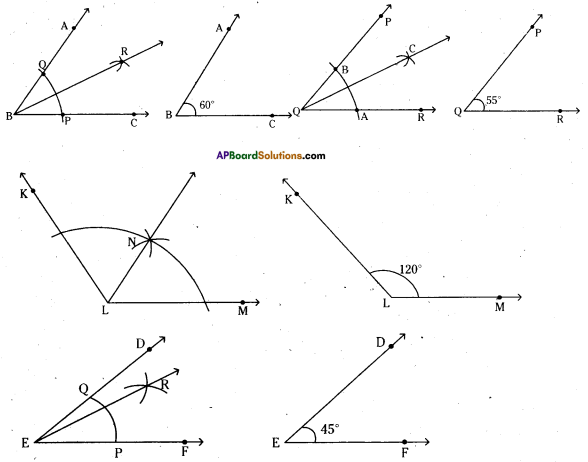AP State Syllabus AP Board 6th Class Maths Solutions Chapter 10 Practical Geometry Ex 10.3 Textbook Questions and Answers.

## AP State Syllabus 6th Class Maths Solutions 10th Lesson Practical Geometry Ex 10.3Question 1.
Construct the following angles with the help of a protractor.
i) ∠ABC = 65°
ii) ∠PQR = 136°
iii) ∠Y = 45°
iv) ∠O = 172°
Solution:
i) ∠ABC = 65°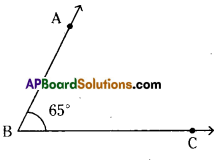Steps of construction :

1. Draw a ray $$\overrightarrow{\mathrm{BC}}$$ of any length.
2. Place the centre point of the protractor at B and the line be aligned with $$\overrightarrow{\mathrm{BC}}$$.
3. Mark a point A at 65°.
4. Join BA. ∠ABC = 65° is formed.
Hence the required angle ∠ABC = 65° is constructed.

ii) ∠PQR = 136°
Steps of construction :

1. Draw a ray $$\overrightarrow{\mathrm{QR}}$$ of any length,
2. Place the centre point of the protractor at Q and the line be aligned with $$\overrightarrow{\mathrm{QR}}$$.
3. Mark a point P at 136°. ‘
4. Join PQ. ∠PQR = 136° is formed.
Hence the required angle ∠PQR = 136° is constructed.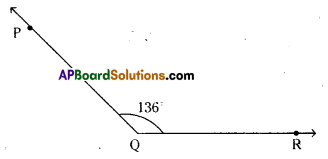iii) ∠Y = 45°
Steps of construction:

1. Draw a ray $$\overrightarrow{\mathrm{YZ}}$$ of any length.
2. Place the centre point of the protractor at Y
and the line be aligned with $$\overrightarrow{\mathrm{YZ}}$$.
3. Mark a point X at 45°.
4. Join YX. ∠XYZ = 45° is formed.
Hence the required angle ∠XYZ = 45° is constructed.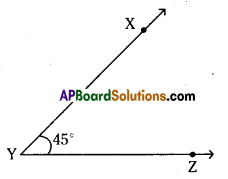iv) ∠O = 172°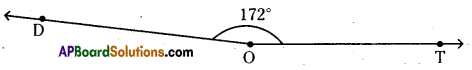Steps of construction:

1. Draw a ray $$\overrightarrow{\mathrm{OT}}$$ °f any length.
2. Place the centre point of the protractor at O and the line be aligned with $$\overrightarrow{\mathrm{OT}}$$
3. Mark a point D at 172°.
4. Join OD. ∠DOT = 172° is formed.
Hence the required angle ∠DOT = 172° is constructed.

Question 2.
Copy the following angles in your note book and find their bisectors: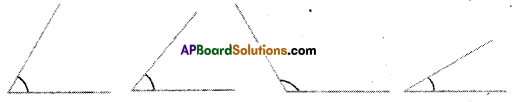Solution: